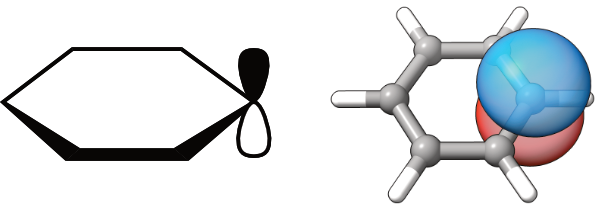# Visualizing atomic type orbitals in molecules

How to compute and visualize natural atomic orbitals with PySCF

quantum chemistry

# Introduction

Sometimes we need to display atomic type orbitals in a schematic way to visualize simple concepts. The molecular orbitals or even localized orbitals are then overly complex. Simple examples are the ChemDraw-style orbitals, which are used to rationalize reactions in organic chemistry. Now, is it possible to obtain similar orbitals, but in 3D?# Computing the 3D structure

We will use benzene as an example. First we generate the 3D coordinates using RDKit

``````from rdkit import Chem
from rdkit.Chem import AllChem

smiles = "c1ccccc1"
mol = Chem.MolFromSmiles(smiles)
mol````````````AllChem.EmbedMolecule(mol)
AllChem.MMFFOptimizeMolecule(mol);``````

We visualize the structure using py3Dmol

``````import py3Dmol

v = py3Dmol.view()
v.setStyle({'stick':{}});``````

# Calculating the NAOs

We will now use PySCF to calculate the NAOs. As we are only interested in the schematic form of the orbitals, the small STO-3G basis set will be sufficient. First we construct the PySCF Mole object from the RDKit Mol object.

``````import pyscf
from pyscf import gto, lo, tools, dft

elements = [atom.GetSymbol() for atom in mol.GetAtoms()]
coordinates = mol.GetConformer().GetPositions()
atoms = [(element, coordinate) for element, coordinate in zip(elements, coordinates)]

pyscf_mole = gto.Mole(basis="sto-3g")
pyscf_mole.atom = atoms
pyscf_mole.build();``````

We then run the DFT calculation, which is actually quite fast

``````mf = dft.RKS(pyscf_mole)
mf.xc = 'b3lyp'
mf.run();``````
``converged SCF energy = -229.251421996489``

We can now compute the NAOs from the 1-st order reduced density matrix. Note that we are here actually calculating the pre-orthogonal NAOs (PNAOs) that are even more local that the NAOs. We the write the PNAOs to cube files - these files can be quite large, ca 3 MB each.

``````dm = mf.make_rdm1()
naos = lo.nao.prenao(pyscf_mole, dm)

for i in range(naos.shape):
tools.cubegen.orbital(pyscf_mole, f'benzene_nao_{i+1:02d}.cube', naos[:,i],  nx=60, ny=60, nz=60)``````

# Visualizing the NAOs

Here we use py3Dmol and ipywidgets to interactively view the orbitals.

``````def draw_orbital(view, i):
with open(f"./benzene_nao_{i:02d}.cube") as f:
view.addVolumetricData(cube_data, "cube", {'isoval': -0.04, 'color': "red", 'opacity': 0.75})
view.addVolumetricData(cube_data, "cube", {'isoval': 0.04, 'color': "blue", 'opacity': 0.75})